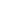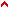1.3.5 Fig 12 - three circles
FIGURE OF THREE CIRCLESIn this figure of three circles, a doctrine is provided for placing circles within one another, where any circle is equivalent in containing capacity to any other, regardless of the fact that one is located inside another.

To measure this figure's equal parts, we follow the method we used in the preceding figure of 3 triangles. It is useful to know the measurements of this figure, as it teaches the way to place one circle inside another so that the contained circle is equivalent in quantity, essence, form and matter to the one containing it.

And the senses can tell that house a. is equivalent to house b., and house b. is equivalent to house c. Further, this figure is useful for essentially and naturally equating the circle with the triangle, given that a. in this figure is equivalent to a. in the figure of three triangles, and the same with b. and c.Top